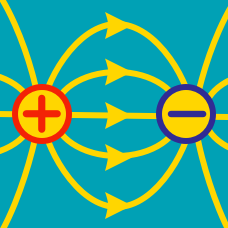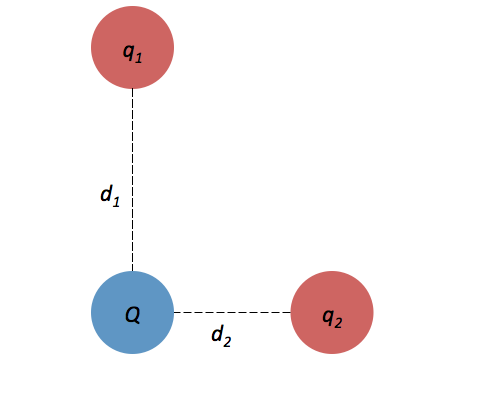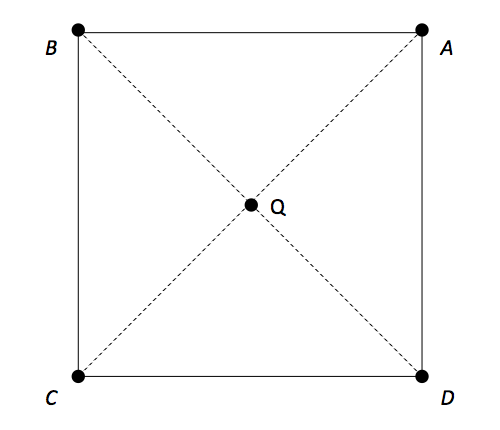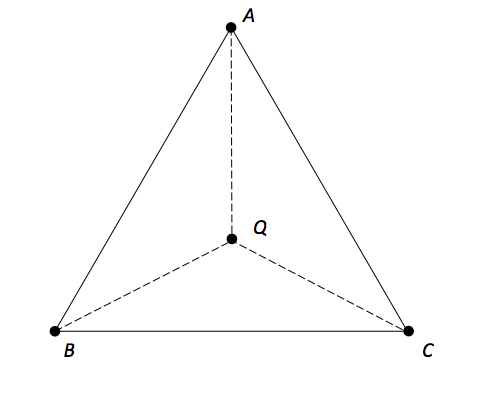Electricity and Magnetism

Superposition of electric fieldsThree point charges of strengths $q_1 = 4.0 \times 10^{-6} \text{ C},$ $q_2 = 1. 0 \times 10^{-6} \text{ C}$ and $Q = -4.0 \times 10^{-6} \text{ C}$ are fixed in a right triangle as shown above.

The distance between $q_1$ and $Q$ is $d_1 = 4.0 \text{ cm},$ and the distance between $q_2$ and $Q$ is $d_2 = 3.0 \text{ cm}.$ What is the approximate electric field strength at location of charge $Q$ due to the other two charges?Four point charges, each equal to $q = 44 \ \mu \text{C},$ are held at the corners of square $ABCD$ of side length $a= 80 \text{ cm}$ on the $xy$-plane. Find the magnitude and sign of a charge $Q$ placed at the center of the square such that the system of charges is in equilibrium.Three point charges, each equal to $q = 33 \ \mu \text{C},$ are held at the corners of equilateral triangle $ABC$ of side length $a= 60 \text{ cm}$ on the $xy$-plane. Find the magnitude and sign of a charge $Q$ placed at the center of the triangle such that the system of charges is in equilibrium.

Two charges, each equal to $-52 \ \mu \text{C},$ are held a certain distance apart. A charge $Q$ is placed exactly midway between them. Find the magnitude and sign of $Q$ such that the system of the three charges is in equilibrium.Two points charges $q_ 1 = 64 \ \mu \text{C}$ and $q_2 = -9 \ \mu \text{C}$ are held on the $x$-axis. The point charge $q_1$ is at $x = -50 \text{ cm}$ and $q_2$ is at the origin. Where on the $x$-axis should a third charge $+Q$ be placed so that charge $Q$ does not experience any net force?

×